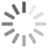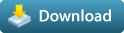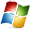Mathematic Nodes (Mentalray Support ) 2.5.2 for Maya (maya plugin)

Mathematic Nodes for Maya and MentalrayRating: 4.7
(15)

Compatibility

• 2012, 2011, 2010, 2009, 2008

Operating Systems

••KeywordsMathematic Nodes for Maya with Support of mentalray

It is set of Mathematic Nodes (over 60 functions) for Maya. It allows you to control your shading Network, Key frame and Procedural Animation, Character or vehicles Rigs, based on Mathematic functions without writing expression. With these nodes you can convert your expression to Maya Standard nodes. This gives you faster evaluation of DG Network (most of the time).

Documentation: https://www.cgdna.com/web/mathematic-nodes-main

Mathematic Nodes DescriptionThe Angular utility node allows you to calculate the value to radian/degree/minutes/seconds.
All the input and output attributes have x, y, and z, components. If you just want to work with single values, then just use one of the x, y, or z channels.

type could be :

1: degree
2: minutes
3: secondsCalculating instant and constant speed of animcurve based on given input time.

type could be :

0: no operation - inputCurve value pass through node with no changes.
1: speed - Returns speed of animcurve based on time.

tip: Connect particular Animcurve.output to inputCurve parameterThis node will return Cardinal and Hyperbolic Cardinal number of input value.
more on Cardinal function at:

type could be:

0: no operation - input value(s) pass through node with no changes
1: sinc (default) - Computes Sinus Cardinal of input value
2: sinc hyperbolic - Computes hyperbolic version of Sinus cardinal numberCondition Range node is similar to Maya standard Condition utility, except its capable of testing the input value if its between specified range or not. If the relationship ( inRange [including Min/Max], inRange [excluding Min/Max], outRange [including Min/Max], outRange [excluding Min/Max]) is true, then the first color or texture is output. If the relationship is false, then the second color or texture is output.

type could be :

0: InRange [include Min/Max] - checks if input is between Min and Max values or its equal to min or max. if ( input >= min && input <= max)
1: InRange [exclude Min/Max] - checks if input is between Min and Max values. if ( input > min && input < max)
2: OutRange [include Min/Max] - checks if input is not in range of Min and Max or its equal to min or max.
3: OutRange [exclude Min/Max] - checks if input is not in range between Min and Max.This node will return pre-defined constant values.

type including:

0: pi (default) - return the Pi (3.141593) value.
1: e - the e (2.718282) value.
2: golden ratio - the golden ratio (1.618034) value.
3: euler gamma - the euler gamme (0.577216) value.
4: degree - the degree (0.0174533) value.
5: catalan - the catalan (0.915966) value.
6: glaisher - the glaisher (1.282427) value.
7: khinchin - the khinchin (2.685452) value.
8: parabolic - the parabolic (2.295587) value.The Curvefunc node lets you make smooth, incrementing transition between values.

type could be :

0: no operation - input value(s) pass through node with no changes

1: linstep (default) - Calculates a value from 0 to 1 that represents a parameter's proportional distance between a min and max value

2: smoothstep - Calculates a value from 0 to 1 that represents a parameter's proportional distance between a min and max value. The smoothstep works like the linstep, except it increases values more quickly near the middle values between the min and max value. The function uses hermite interpolation between min and max value.

3: polynominal hermite - returns the value of the Hermite Polynomial of order n at point x. (45 is the maximum for curve order)The Exponential Node lets you calculate some exponential values including exp, log, log10, sqrt, cbrt. type could be :

0: no operation - input value(s) pass through node with no changes

1: exp (default) - Calculates 'e' raise it to the power of a number, e^number. The predefined variable 'e' is the base of the natural logarithm, which is 2.718.

2: log - Calculates the natural logarithm of a number, logenumber. the natural logarithm uses the constant 'e', which is 2.718.

3: log10 - Calculates the log base 10 of a number.

4: sqrt - Calculates the square root of a positive number.

5: cbrt - Computes cubed root of input value.This node will compute Rising factorial number of Base input. more on Factorial function at:

https://mathworld.wolfram.com/Factorial.html

type could be :

0: no operation - input value(s) pass through node without changes
1: factorial (default) - Computes Rising factorial of Base value.The Limit Node is a math function that imposes limits on values.

type could be :

0: no operation - input value(s) pass through node with no changes

1: abs - (default) - Calculates the absolute value(s) of input. The absolute value of an integer or floating point is the number without its positive or negative sign.

2: ceil - Calculates a value rounded to the smallest integer value greater that or equal to a floating point number.

3: floor - Calculates a value rounded to the largest integer less than o equal to a floating point number.

4: sign - Calculates one of three values representing the sign of a value. Return -1 if the number is negative, 1 if positive, 0 if 0.

5: trunc - Returns whole number part of a floating point number.

6: fraction - Returns fraction part of floating point number.

7: round - Returns a number rounded to a specified number of decimal places.Returns Minimum or Maximum value of animcurve keyframes.

type could be :

0: no operation - inputCurve value pass through node without any changes.
1: min - returns Minimum value of inputCurve key frames.
2: max - returns Maximum value of inputCurve key frames.

tip: Connect particular Animcurve.output to inputCurve parameterThe Limit Min Max Node finds minimum and maximum of value(s).

This node actually has different input attributes for using different kinds of data. For simple numbers, you use the Input1D attribute and get the result from Output1D. For 2d attributes, such as UV coordinates, you use the Input2D attribute and get the result from Output2D. For 3d attributes, like vectors or colors or 3d coordinates, you use the Input3D attribute and get the result from the Output3D attribute.

type could be :

0: no operation - input value(s) pass through node without any changes.

1: min (default) - find minimum value(s) of input

2: max - find maximum value(s) of inputThis utility can solve general form of 2nd degree Polynomial equation, including finding possible roots, delta and vertex of the equation.

f(x) = ax2 + bx + c , where a=! 0;

The graph of this quadratic function is Parabola whose major axis is parallel to the y-axis.

note: if the equation doesnt have roots, for sake of compatibility it will return Zero for roots.
more on Parabolic at:This node will return True Gamma (Tgamma), Logarithmic Gamma (Lgamma) and Logarithmic Derivate Gamma (Digamma) of Base input.

more on Gamma functions at:

type could be :

0: no operation - input value(s) pass through node without changes
1: Tgamma (default) - computes true gamma of base value
2: Lgamma - computes the natural logarithm of the absolute value of the gamma function of base value.
3: Digamma - compute the logarithmic derivative of the gamma functionThe Trigonometric Node returns trigonometric value(s) or input, including sine, sine hyperbolic, arc sine, cosine, cosine hyperbolic, arc cosine, tangent, tangent hyperbolic, arc tagent

type could be :

0: no operation - input value(s) pass through node without any changes
1: sin (default)
2: sin hyperbolic
3: arc sin
4: cos
5: cos hyperbolic
6: arc cos
7: tan
8: tan hyperbolic
9: arc tan

10: hypot - The hypot function returns the length of the hypotenuse of a right triangle. Specify the lengths of the sides adjacent to the right angle as X and Y.

Please use the Bug System to report any bugs.
Please use the Feature Requests to give me ideas.
Please use the Support Forum if you have any questions or problems.
Please rate and review in the Review section.